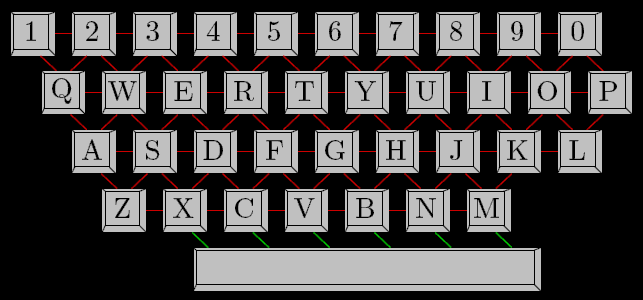### Problem Statement

An application you're working on contains an input text field where a user types in a date.

The user is instructed to type in a date in the form "<Month> <Day>" (quotes for clarity), where <Month> is the English name of the month ("January", "February", etc.) and <Day> is the day of the month, without leading zeroes.

However, a user can make errors when typing. The following model is suggested: the user always presses the correct number of keys, but he or she can mistype one key for another. Let's define the penalty for a typing error as the length of the shortest path between the key that was actually pressed and the intended key in the graph shown below.Here red lines denote edges of length 1 and green lines denote edges of length 3. When calculating the penalty, the cases of the letters are ignored.

Obviously, the penalty for typing a correct key is 0. Now, define the distance between the input of the user and a correct date of the same length as the sum of the penalties for mistyping over all corresponding characters.

For example, distance("TopCoder", "March 31") = penalty("T", "M") + penalty("O", "A") + ... + penalty("R", "1") = 4 + 8 + 6 + 0 + 3 + 4 + 1 + 4 = 30

Given a String input, return a correct date (in the format described above) that has the smallest distance from the input. In case of a tie, return the date that is earlier in the year.

### Definition

 Class: DateFieldCorrection Method: correctDate Parameters: String Returns: String Method signature: String correctDate(String input) (be sure your method is public)

### Notes

-Consider February 29 a valid date (as it is in leap years).
-The names of the months are "January", "February", "March", "April", "May", "June", "July", "August", "September", "October", "November" and "December".
-The lengths of the corresponding months are 31, 29, 31, 30, 31, 30, 31, 31, 30, 31, 30 and 31 days.

### Constraints

-input will contain between 5 and 12 characters, inclusive.
-input will contain only uppercase and lowercase letters ('A'-'Z', 'a'-'z'), digits ('0'-'9') and spaces (' ').

### Examples

0)

 `"Novebmer 10"`
`Returns: "November 10"`
 Swapping "b" and "m" is just a little typo and should be corrected easily.
1)

 `"September 15"`
`Returns: "September 15"`
 A date that is typed in correctly shouldn't be changed at all.
2)

 `"Juny 4"`
`Returns: "June 4"`
 "Juny" could stand both for "June" and "July". The penalty for mistyping is 3 in each case. If that's the case, June is preferred because it is earlier than July.
3)

 `"Juny 31"`
`Returns: "July 31"`
 Once again, both June and July could have been meant by the user. However, if it's June then there is at least one typo in the day (there is no 31th day in June).
4)

 `"TopCoder"`
`Returns: "April 24"`

#### Problem url:

http://www.topcoder.com/stat?c=problem_statement&pm=8267

#### Problem stats url:

http://www.topcoder.com/tc?module=ProblemDetail&rd=10794&pm=8267

darnley

#### Testers:

PabloGilberto , Olexiy , ivan_metelsky , ged

#### Problem categories:

Graph Theory, Simple Search, Iteration